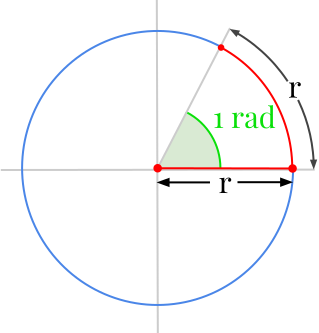👋 Hello! I'm Alphonsio the robot. Ask me a question, I'll try to answer.

# How is defined one radian?

The radian is the SI unit for measuring circles. One radian is defined by the angle of an arc whose length is equal to the radius of this arc.One radian is also numerically equal to ﻿﻿ .

More